# Constructing the Projections of Plane Figures and the Method of Revolution

The projections of a polygon contained in the plane in a general position are also polygons with the same number of sides and vertices. But if a polygon belongs to a projecting plane, then one of its projections is a line segment. With previous knowledge of Monge's method, we are able to construct projections of these latter polygons, and determine their true size.

Assignment 1: Determine the true size of the triangle ABC that belongs to the horizontal projecting plane.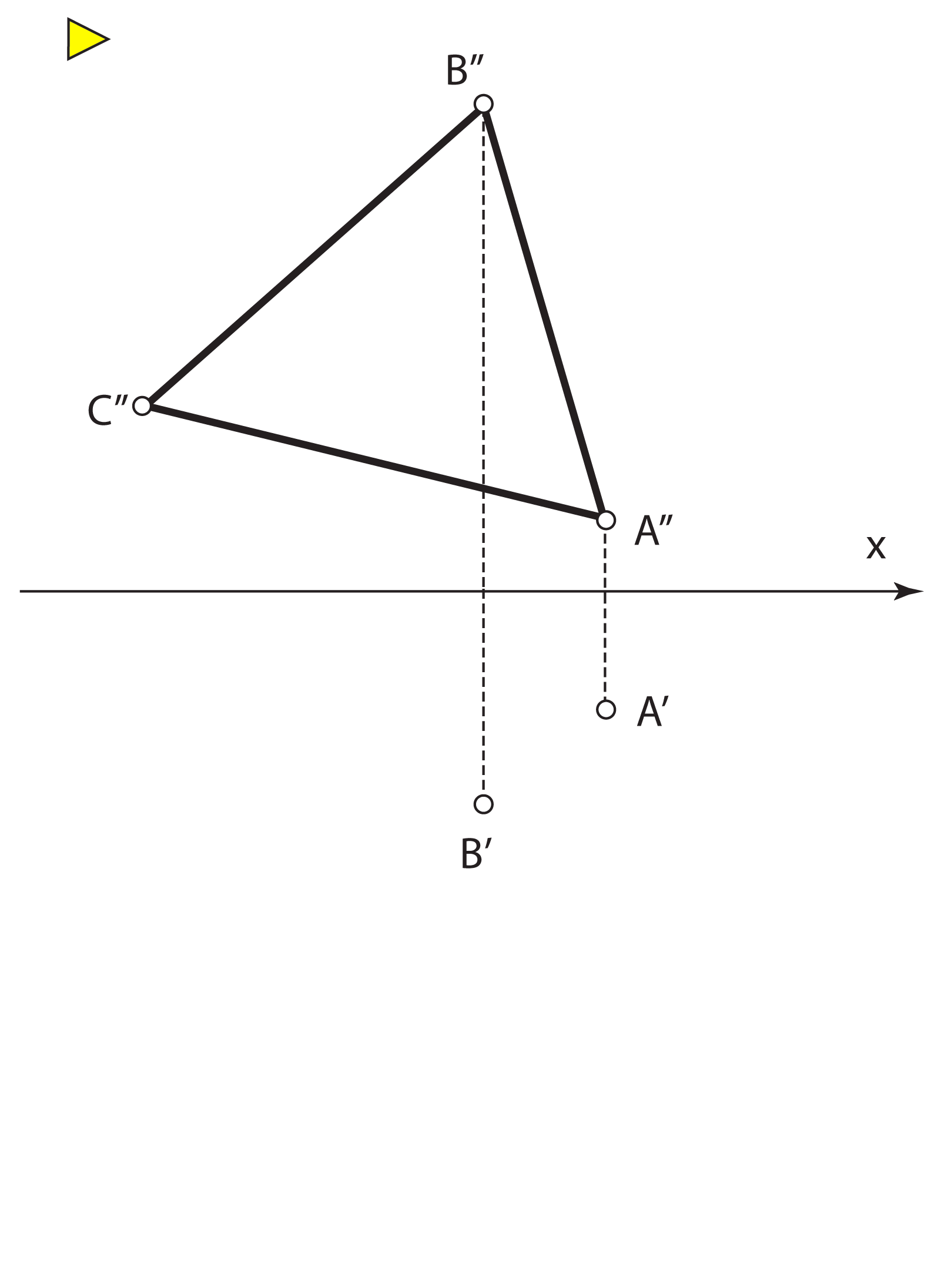Construction of the top view of the point C is based on the fact that the triangle belongs to the horizontal projecting plane so its horizontal projection must be a line u. We rotate the points A, B and C about the horizontal trace into Π1 and in that way obtain the points Ao, Bo and Co. You should already be familiar with this procedure; it is called a revolution of a horizontal projecting plane. Construct the triangle AoBoCo that is congruent to the triangle ABC.

Assignment 2: Determine the true size of the paralellogram ABCD that belongs to a vertical projecting plane.

However, if the polygon is contained in the plane in general position, we cannot construct a polygon congruent to it using this method. Try to solve the next task:

Assignment 3: Determine the true size of a triangle ABC that belongs to the plane P.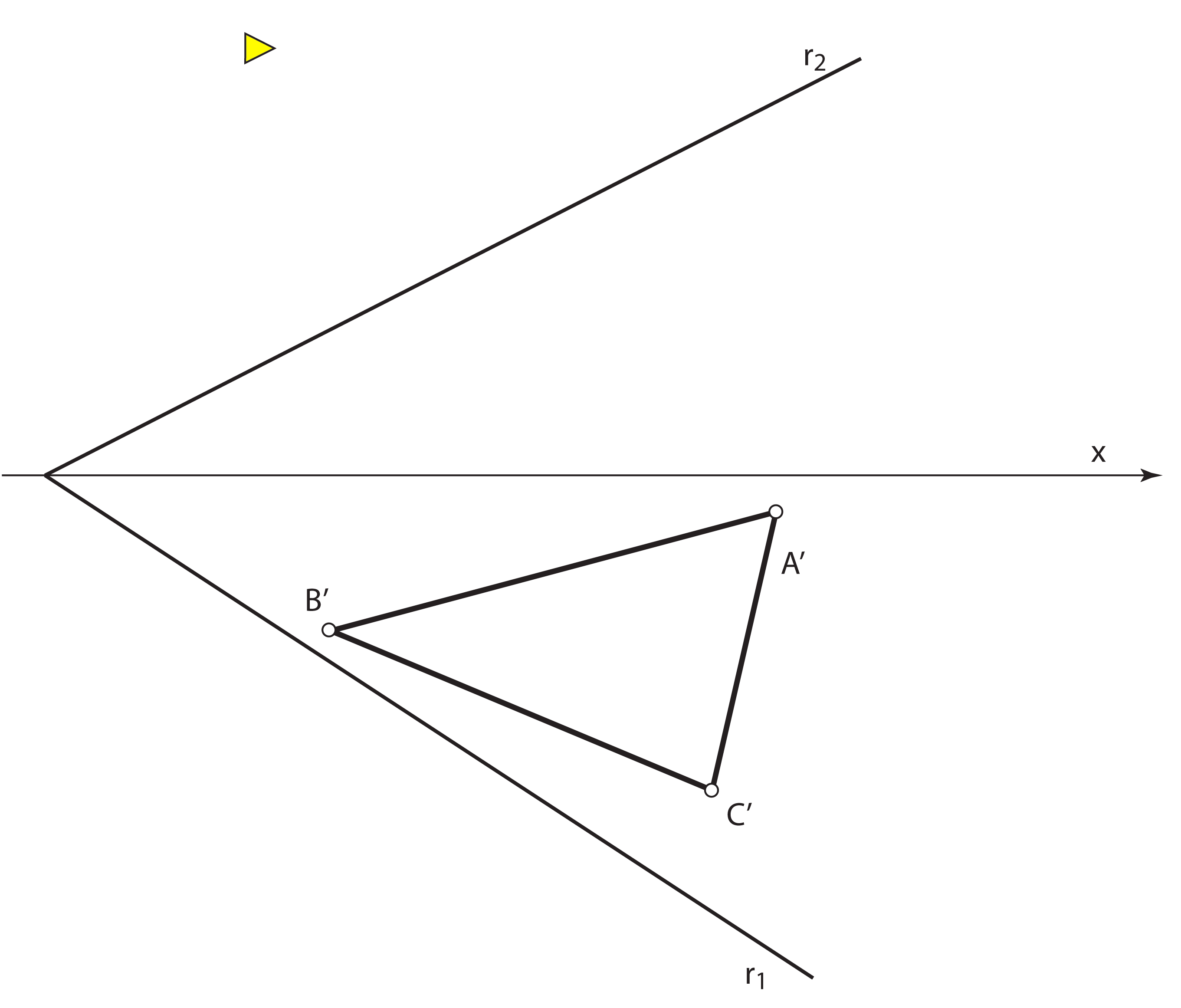We obtain the top view of the triangle using the fact that it lies in the plane P – using horizontal principal lines. To continue solving the assignment, we need to rotate the plane P into one of the planes of projections.

## Revolution of a plane about its trace (method of coincidence)

The plane P is rotated into the plane Π1 about its  horizontal trace (it is brought into coincidence with the horizontal plane of projection) or into the plane Π2 about its vertical trace (it is brought into coincidence with the vertical plane of projection).

CONSTRUCTION  of the projection of a point (the revolution about the horizontal trace).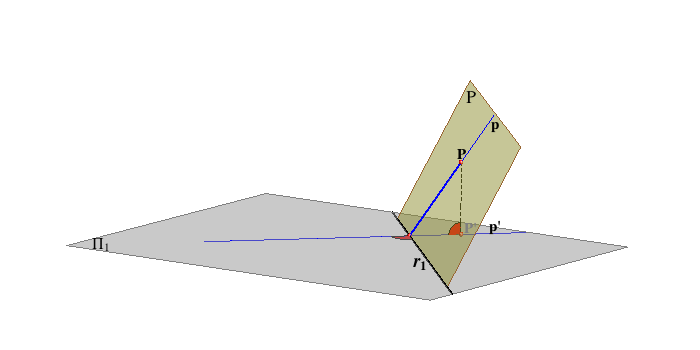Click on the image to start the animation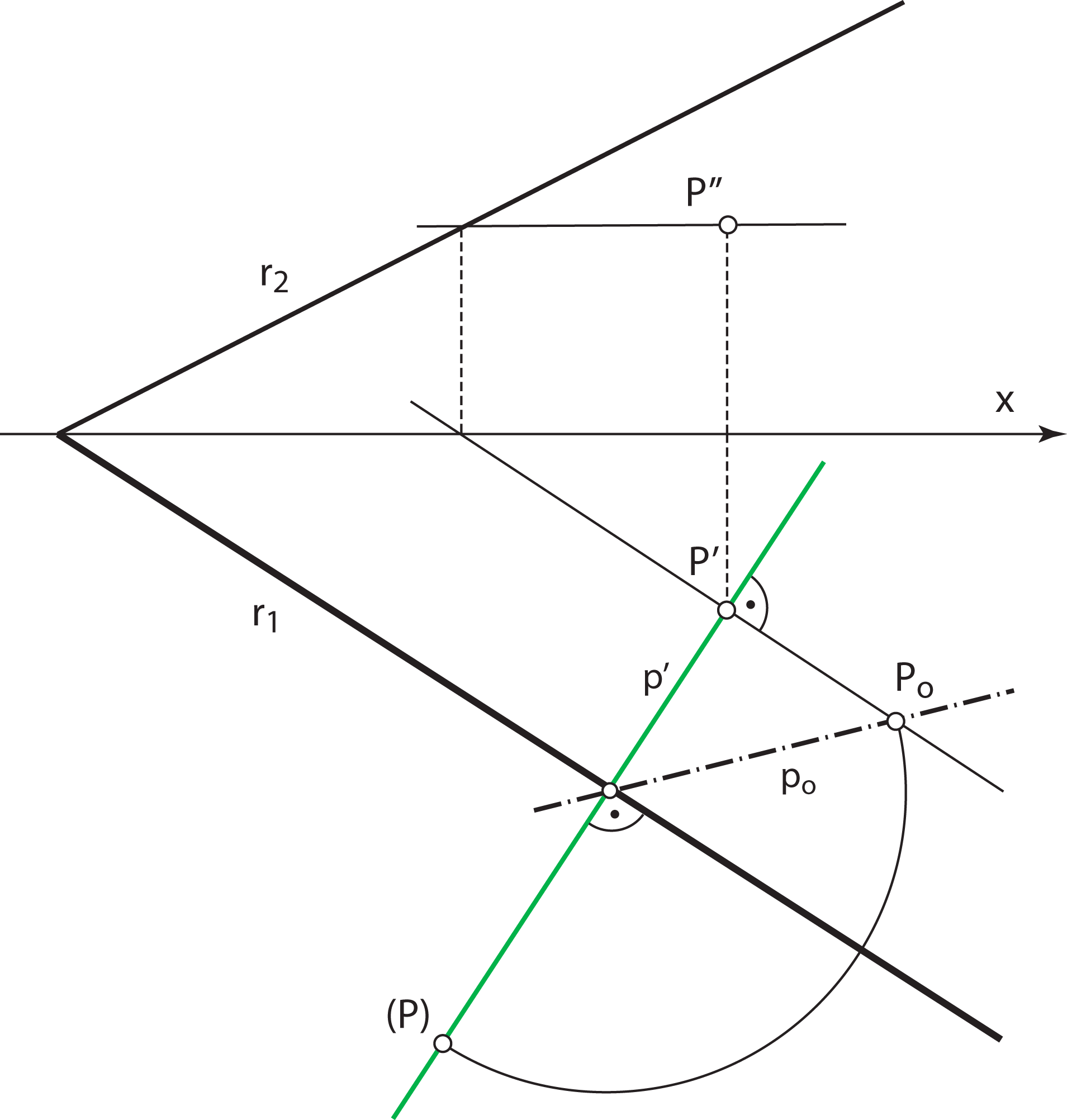We now rotate a triangle.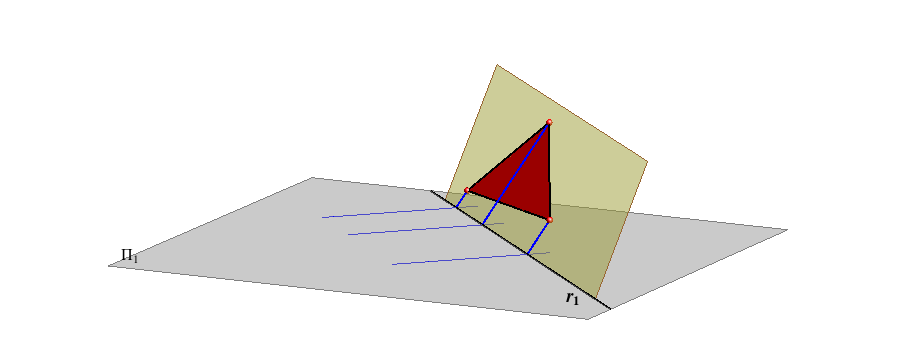Click on the image to start the animation.

Determining the true size of the triangle from Assignment 3.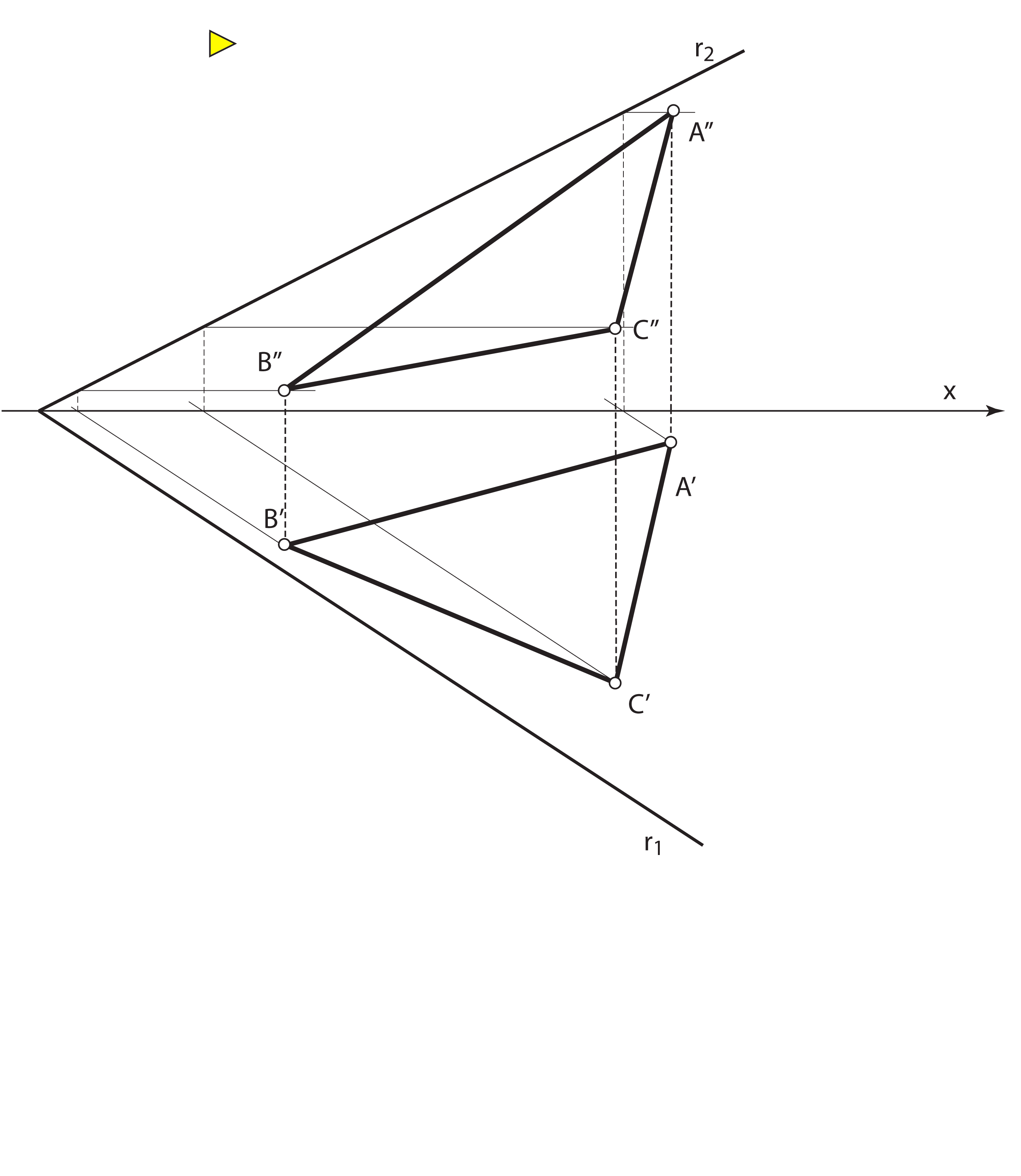Rotate the point A into Π1 about the horizontal trace of the plane P. The image of the point (A) lies on the horizontal projection of the 1st steepest line of the plane P that passes through the point A. A radius of revolution of the point A equals its distance to the trace r1. Notice the following: Horizontal projections of points and lines of the plane P are mapped into their rotated positions in the plane Π1 by an affine transformation. An axis of this transformation is the trace r1, rays are horizontal projections of the 1st steepest lines of the plane P. Using the affine transformation (A',(A),r1) construct the points (B) and (C). Triangle (A)(B)(C) is congruent to the triangle ABC.

The same conclusions are valid if we rotate the plane about its vertical trace. Then we have an affine transformation that maps the vertical projections of points and lines and their rotated positions in the plane Π2. Axis of this transformation is the vertical trace of the plane P, and the rays are vertical projections of the 2nd steepest lines of that plane.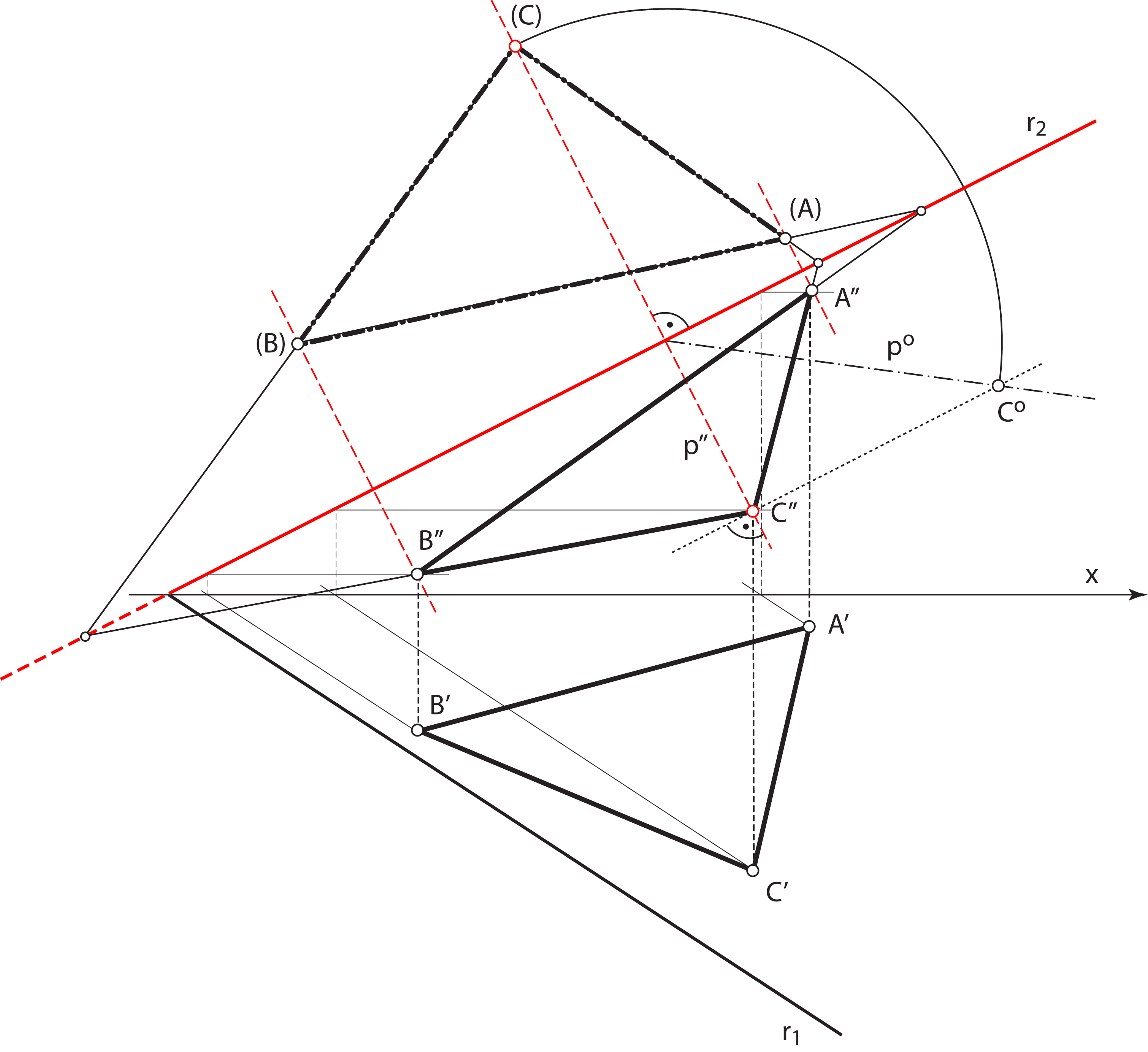## Projections of a circle

If a circle k belongs to the plane in general position P, its orthogonal projection to the projection plane Π is an ellipse k'.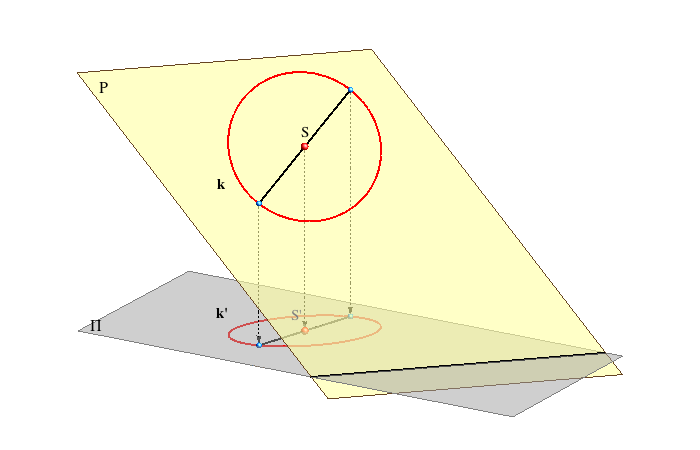Click on the image to start the animation As the parallel lines and ratio of the line lengths are preserved in the orthogonal projection, so the following holds: A center of the circle k is projected into the center of the ellipse k'. Diameters of the circle are projected into diameters of the ellipse. Pairs of orthogonal diameters of the circle are projected into pairs of conjugate diameters of the ellipse. QUESTION: Which pair of orthogonal diameters of the circle k projects into the major and minor axis of the ellipse k'?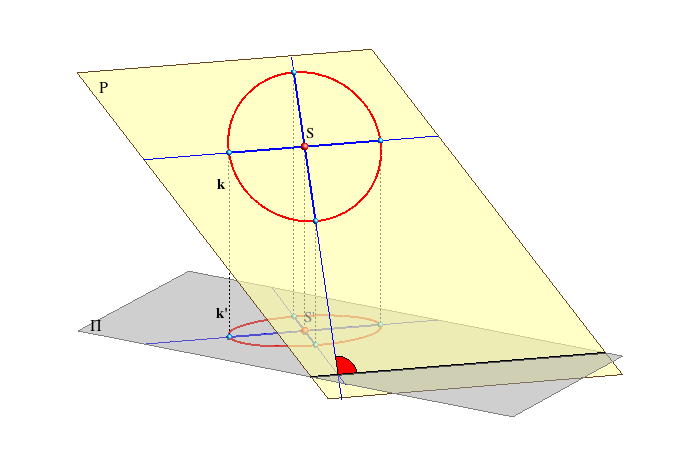A length of the orthogonal projection of a line segment is smaller or the same (if the line segment is parallel to the plane of projection) than the true length of the line segment. Therefore, the diameter of the circle which is parallel to the plane of projection is projected into the longest diameter of the ellipse - its major axis.  The minor axis is perpendicular to the major axis, so its projection lie on a line perpendicular to the projection of the major axis. This line has the largest angle of inclination to the plane of projection. Projections of the line segments on this line have the most reduced lengths. We conclude: A major axis of the orthogonal projection of the circle lies on the principal line of the plane that passes through the projection of the center of the circle. Its length equals the length of the diameter of the circle. A minor axis of the orthogonal projection of the circle lies on the steepest line of the plane that passes through the projection of the center of the circle.

Assignment: Construct the projections of the circle k that lies in a given plane P, if S is its center, and r its radius.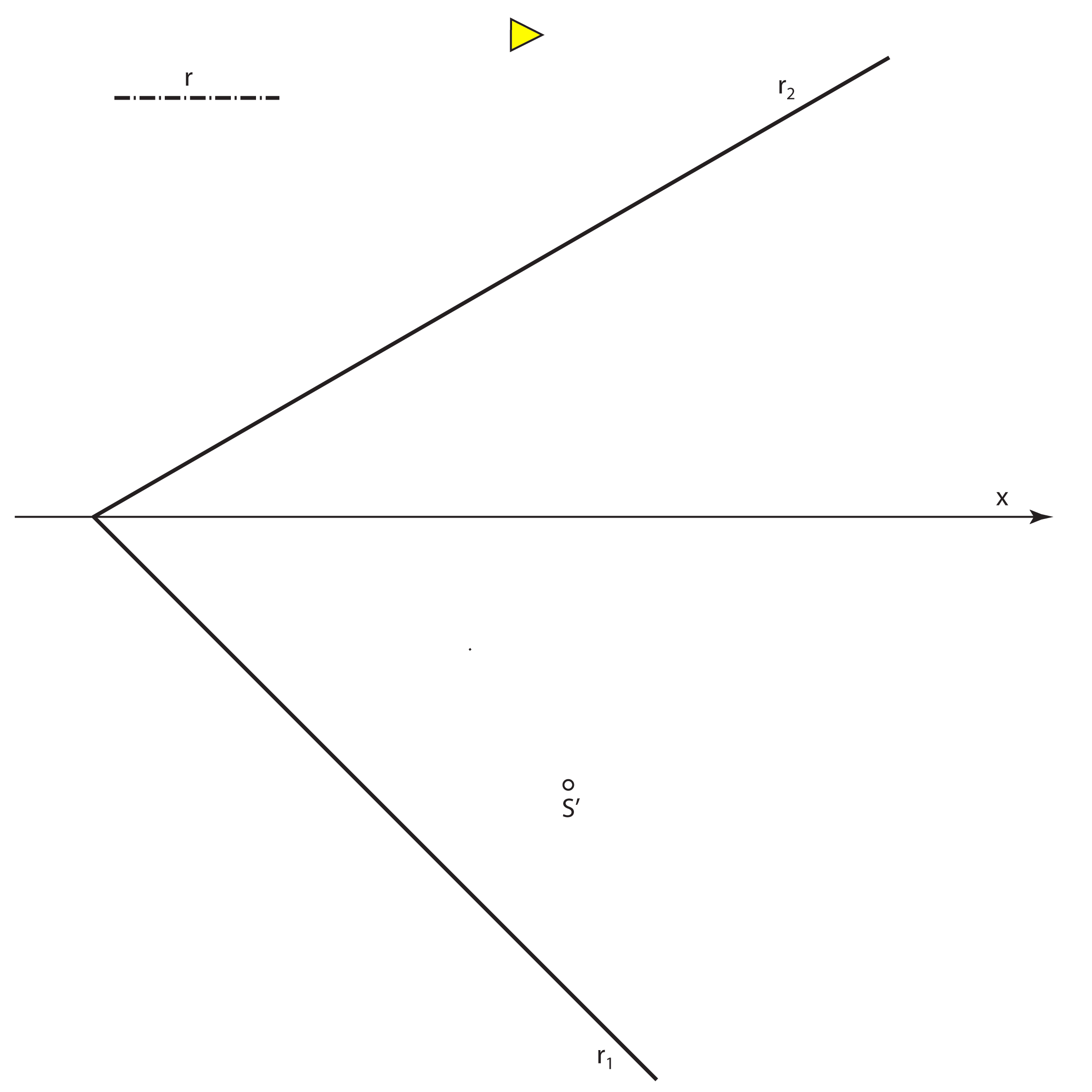A major axis of the vertical projection of the circle k (a major axis of the ellipse k''), lies on the vertical projection of the vertical principal line of the plane P that passes through S'', its length equals 2r. A minor axis of the vertical projection of the circle  k (a minor axis of the ellipse k''),  lies on the vertical projection of the 2nd steepest line of the plane P that passes through S''. Construction : rotate this 2nd steepest line into the plane Π2 and lay the length 2r on the rotated line. The same holds for the construction of the major and minor axis of the horizontal projection of the circle. A major axis of the horizontal projection of the circle k (a major axis of the ellipse k'), lies on the horizontal projection of the horizontal principal line of the plane P that passes through S', its length equals 2r.  A minor axis of the horizontal projection of the circle  k (a minor axis of the ellipse k'),  lies on the horizontal projection of the 1st steepest line of the plane P that passes through S'. Construction : rotate this 1nd steepest line into the plane Π1 and lay the length 2r on the rotated line.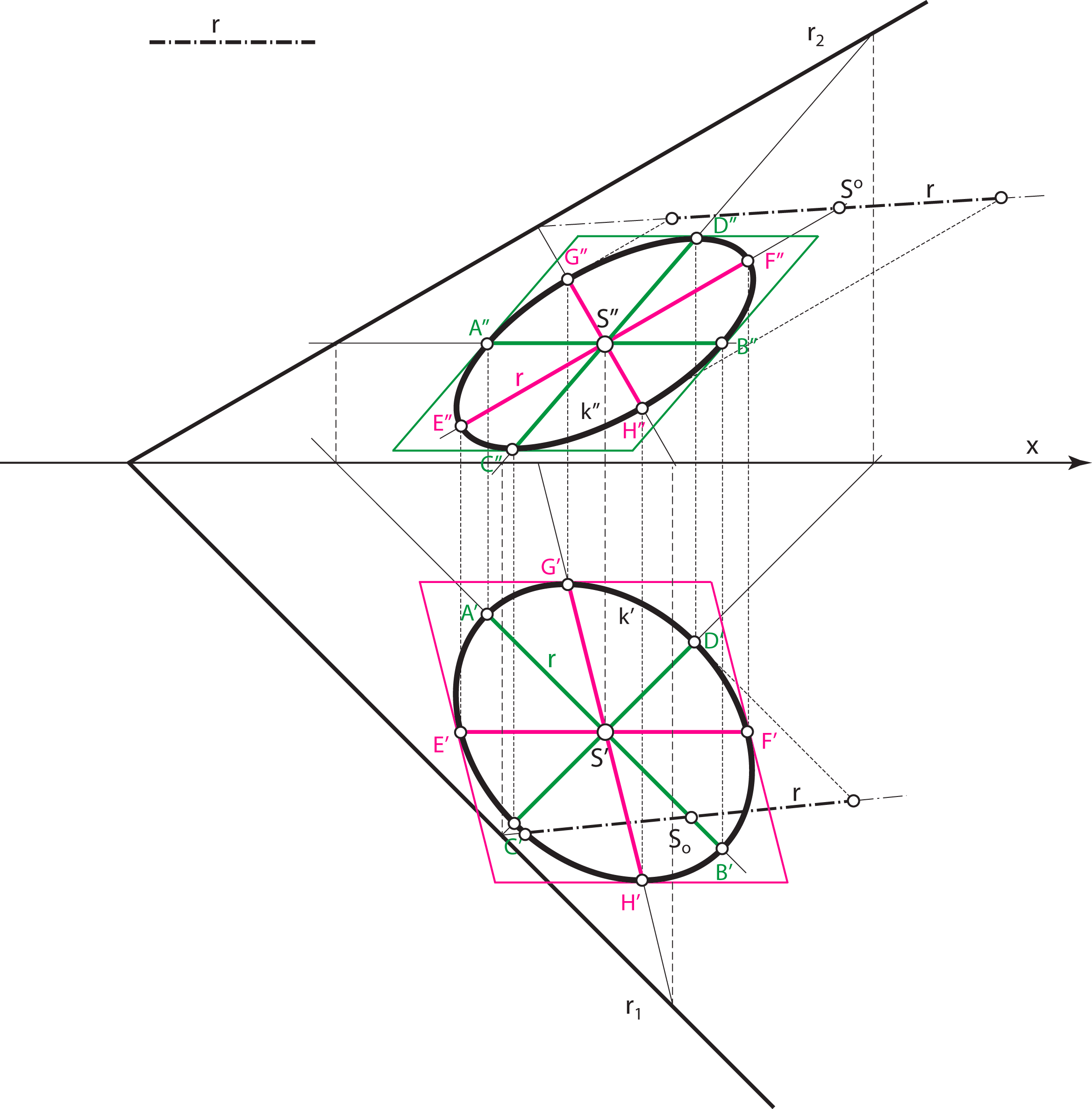A vertical projection of the diameters of the circle k, whose the horizontal projection are the major and the minor axis of the ellipse k' (AB i CD), is a pair of conjugate diameters of the ellipse k''. A horizontal projection of the diameters of the circle k, whose the vertical projection are the major and the minor axis of the ellipse k'' (EF i GH), is a pair of conjugate diameters of the ellipse k'.

• The ellipse k' is the affine transformation of the circle (k) in the plane Π1, the ellipse k'' is the affine transformation of the circle ((k)) in the plane Π2.

### Special positions of the circle

• If a circle lies in a projecting plane, than the corresponding projection of the circle is a line segment, whose length equals the diameter of the circle.

EXAMPLE 1: Projections of a circle that lies in the horizontal projecting plane.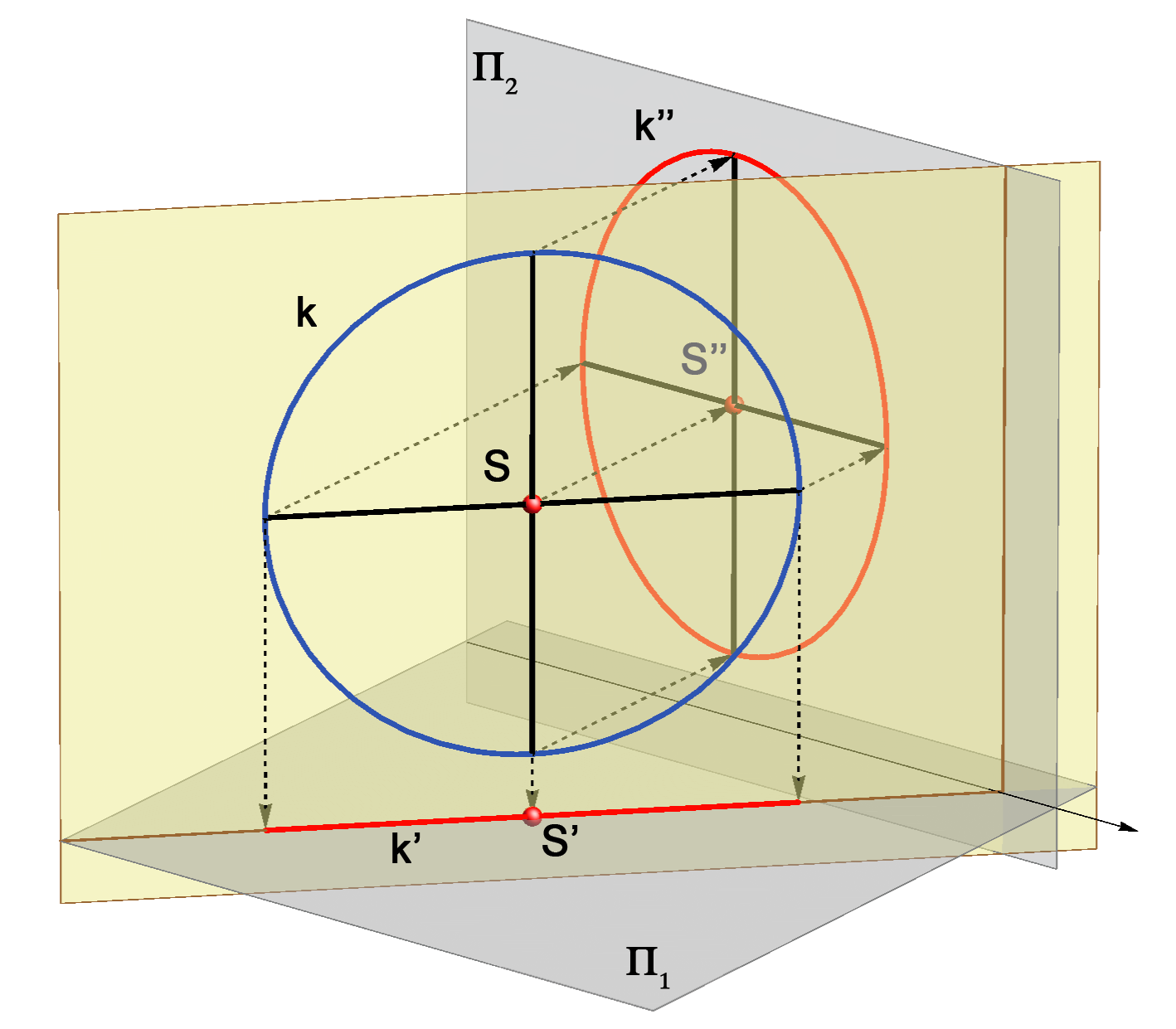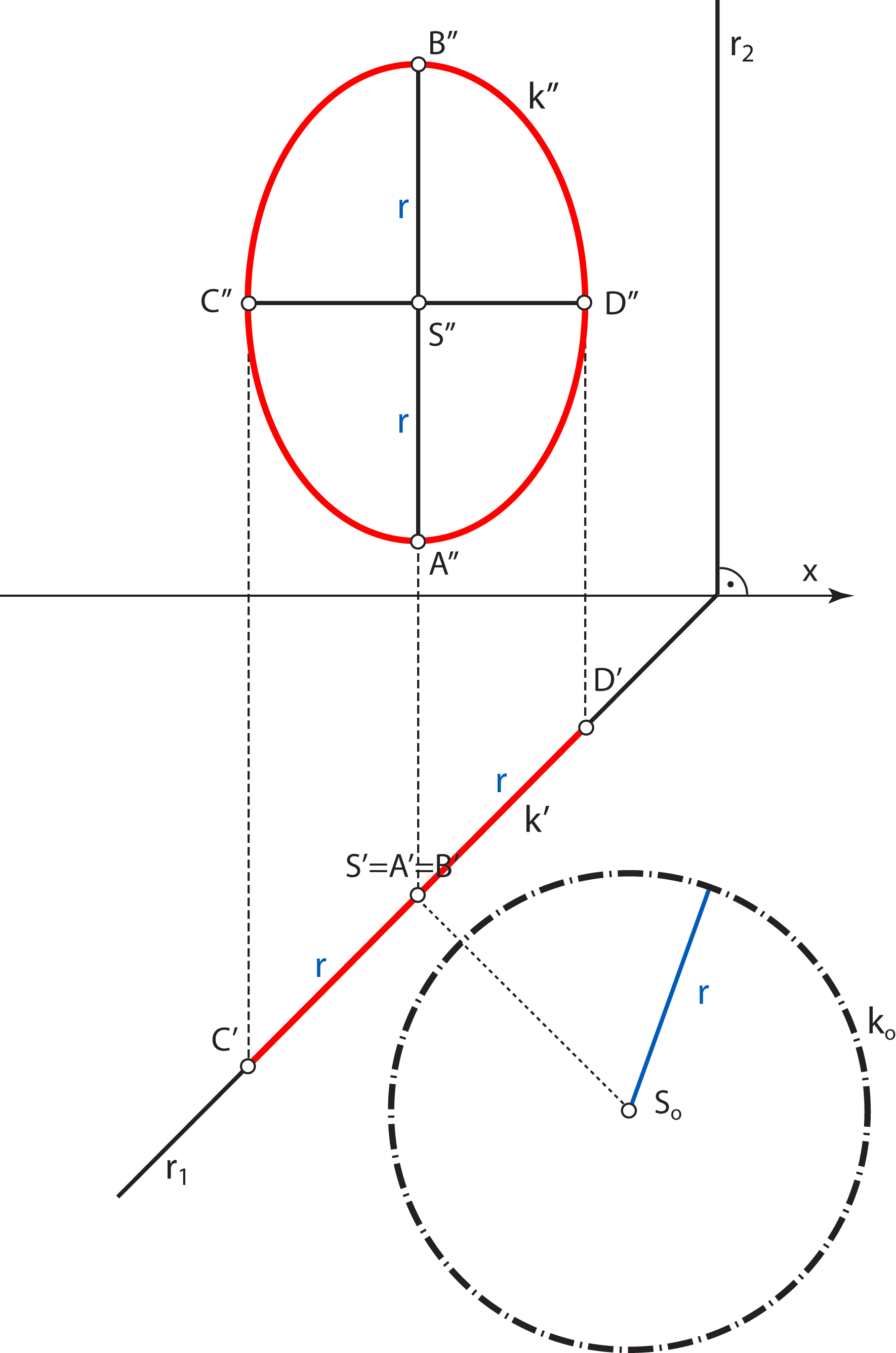EXAMPLE 2: Projections of a circle that lies in the vertical projecting plane.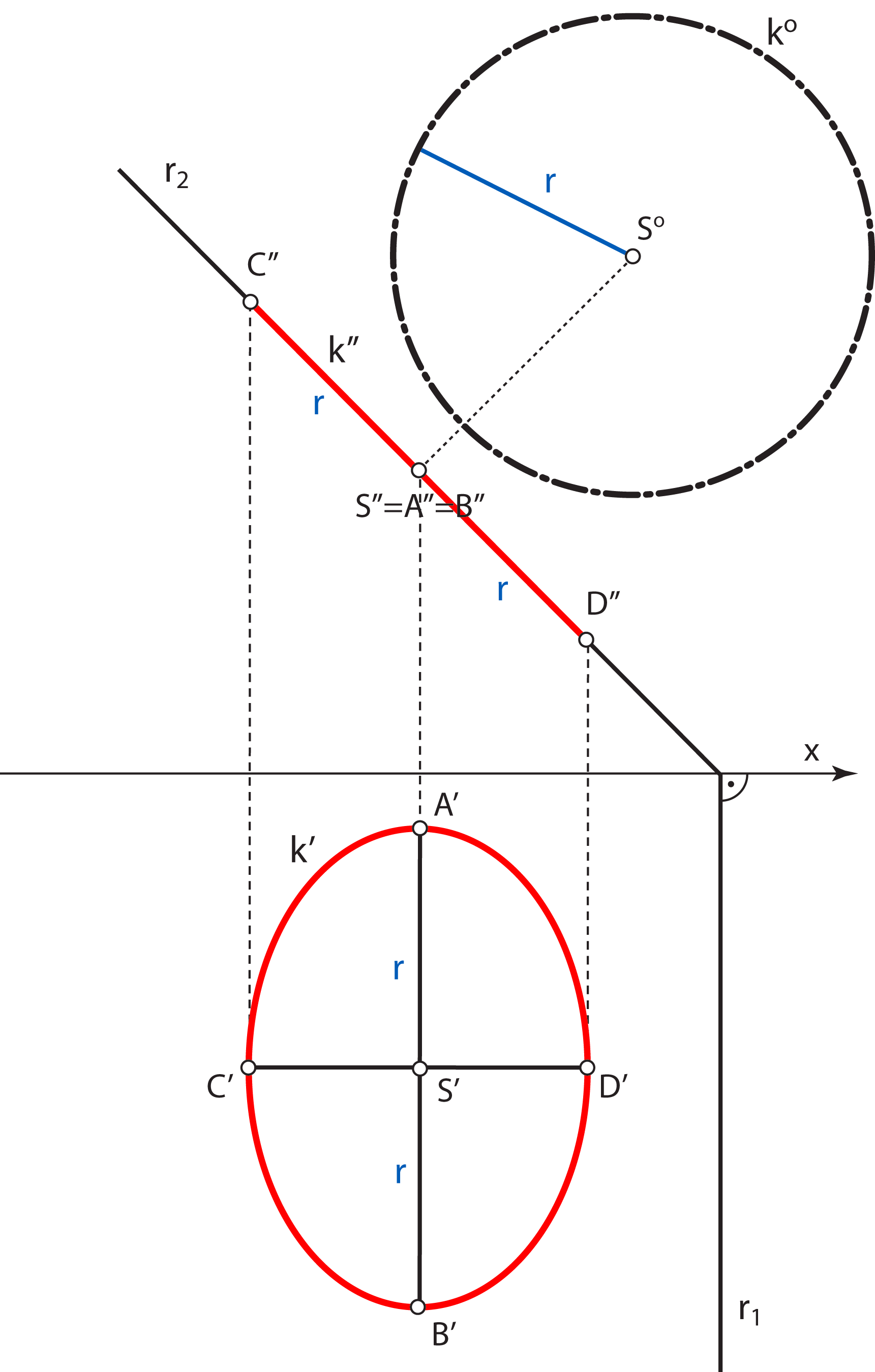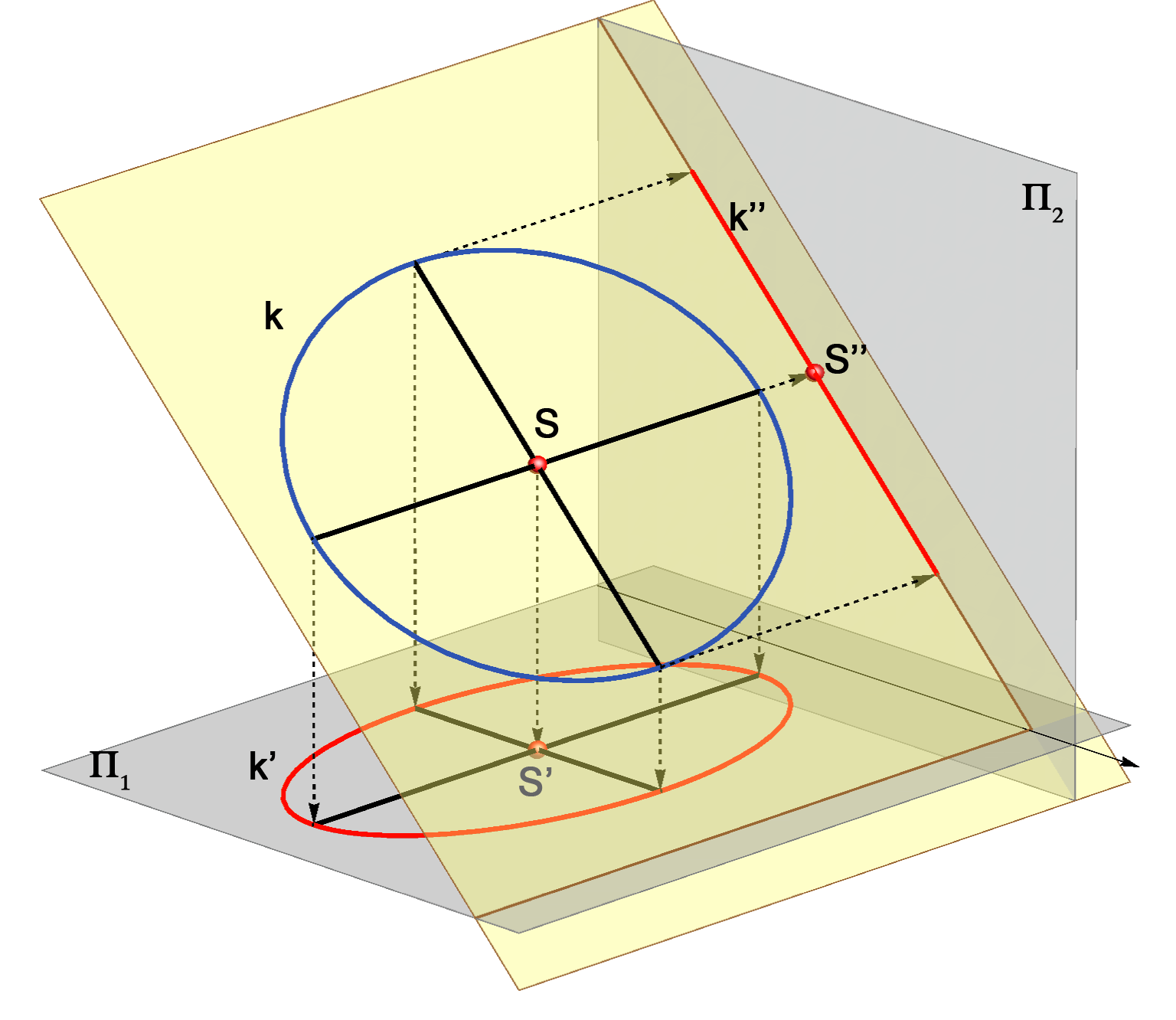• If a circle lies in a plane parallel to one of the planes of projections, its projection to that plane is a circle.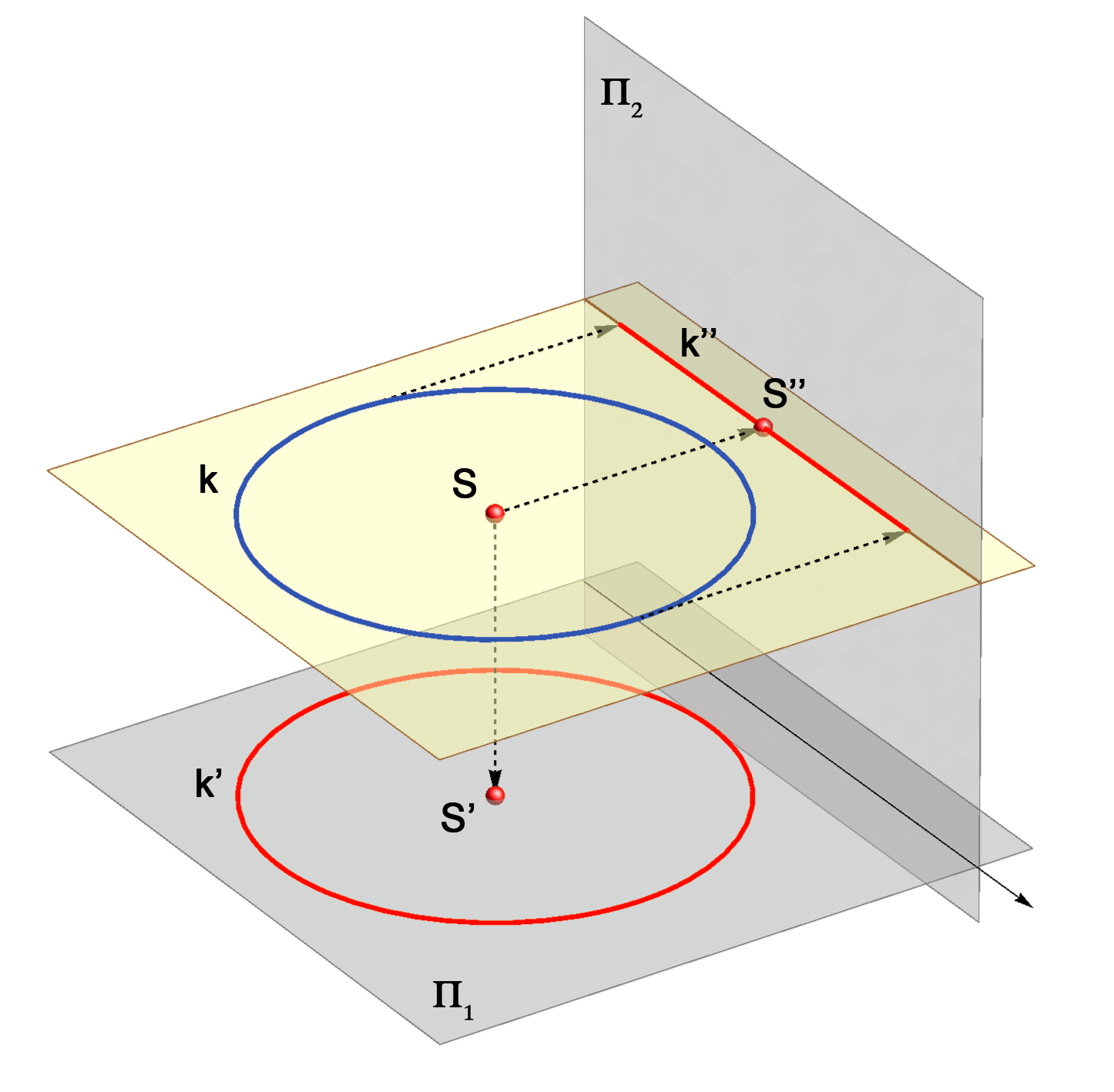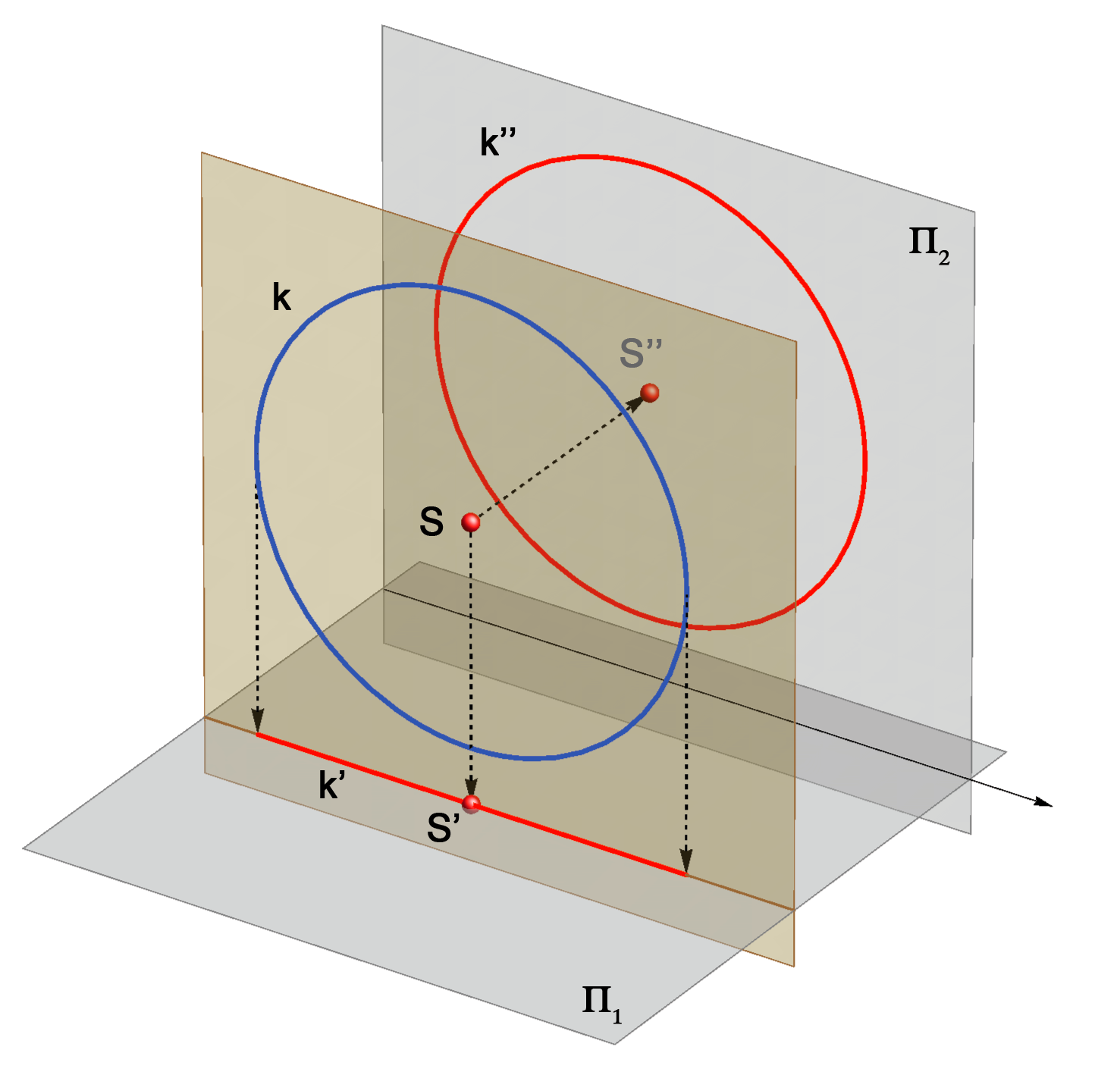Created by Sonja Gorjanc, translated by Helena Halas and Iva Kodrnja -3DGeomTeh - Developing project of the University of Zagreb